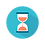From: Bayesian Models for Astrophysical Data, Cambridge Univ. Press

(c) 2017,  Joseph M. Hilbe, Rafael S. de Souza and Emille E. O. Ishida

you are kindly asked to include the complete citation if you used this material in a publication

Code 7.14 Bayesian log-gamma–logit hurdle model in Python using Stan

============================================================

import numpy as np
import pystan
import statsmodels.api as sm

from scipy.stats import uniform, bernoulli

# Data
np.random.seed(33559)                                                           # set seed to replicate example
nobs= 2000                                                                              # number of obs in model

x1 = uniform.rvs(loc=0, scale=2.5, size=nobs)
xc = 0.6 + 1.25 * x1                                                                # linear predictor, xb
y = np.random.lognormal(sigma=0.4, mean=np.exp(xc))

xb = -3.0 + 4.5 * x1                                                                # construct filter
pi = 1.0/(1.0 + np.exp(-xb))
bern = [bernoulli.rvs(1-pi[i]) for i in range(nobs)]

ly = [y[i] * bern[i] for i in  range(nobs)]                                # Add structural zeros

X = np.transpose(x1)

mydata = {}                                                                          # build data dictionary
mydata['Y'] = ly                                                                    # response variable
mydata['N'] = nobs                                                               # sample size
mydata['Xb'] = X                                                                  # predictors
mydata['Xc'] = X
mydata['Kb'] = X.shape                                                   # number of coefficients
mydata['Kc'] = X.shape

# Fit
stan_code = """
data{
int<lower=0> N;
int<lower=0> Kb;
int<lower=0> Kc;
matrix[N, Kb] Xb;
matrix[N, Kc] Xc;
real<lower=0> Y[N];
}
parameters{
vector[Kc] beta;
vector[Kb] gamma;
real<lower=0> sigmaLN;
}
model{
vector[N] mu;
vector[N] Pi;

mu = exp(Xc * beta);

for (i in 1:N) Pi[i] = inv_logit(Xb[i] * gamma);

for (i in 1:N) {
(Y[i] == 0) ~ bernoulli(Pi[i]);
if (Y[i] > 0) Y[i] ~ lognormal(mu[i], sigmaLN);
}
}
"""

# Run mcmc
fit = pystan.stan(model_code=stan_code, data=mydata, iter=7000, chains=3,
warmup=4000, n_jobs=3)

# Output
print(fit)

============================================================Output on screen:

Inference for Stan model: anon_model_c491b597a93174aa69fa4bfeba9aa6bd.
3 chains, each with iter=7000; warmup=4000; thin=1;
post-warmup draws per chain=3000, total post-warmup draws=9000.

mean     se_mean            sd         2.5%       25%        50%         75%      97.5%      n_eff       Rhat
beta               0.59        1.2e-4       8.7e-3        0.57        0.58         0.59           0.6         0.61       4958         1.0
beta               1.26        1.3e-4       8.8e-3        1.24        1.25         1.26         1.26         1.27       4829         1.0
gamma         -3.09        2.5e-3         0.17        -3.42      -3.19        -3.08        -2.97        -2.76       4425        1.0
gamma          4.55        3.1e-3         0.21          4.15       4.41         4.54          4.68         4.97       4395        1.0
sigmaLN            0.42       1.6e-4          0.01           0.4        0.41         0.42          0.43         0.44       5687        1.0
lp__                -349.7           0.02         1.47      -353.4     -350.5      -349.4      -348.7      -347.8       4138        1.0

Samples were drawn using NUTS at Mon May  1 00:36:55 2017.
For each parameter, n_eff is a crude measure of effective sample size,
and Rhat is the potential scale reduction factor on split chains (at
convergence, Rhat=1).